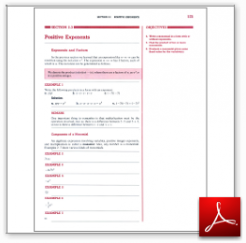Algebra Math Book - Introductory Algebra Thank you for your continual support.

 The power of mathematics rests in the logic of thinking.ID: Sec2-3
Description: Exponents and Factors-Monomials-Multiplying Monomials-Evaluating a Monomial
Price: 1.99

# Algebra Math book - Introductory Algebra - Chapter 2 - Section 3 - Positive Exponents

## Section 2.3 - Objectives

4.  Write a monomial in a form with or without exponents.

5.  Find the product of two or more monomials.

6.  Evaluate a monomial given some fixed value for the variable(s).

Navigate to
Previous Section or Next Section
or Chapter 2 Details or Other Chapters

This section of my algebra math book, Introductory Algebra, also includes in the download:

• Cover Sheet